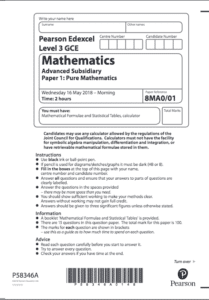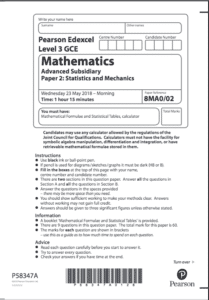# AS Maths (first year of A-Level Mathematics)

AS Maths is the name we give to the first year of A-Level Mathematics. The ‘AS’ stands for ‘Advanced Subsidiary’.  Students can study for 1 year and take exams to get a qualification in AS-Level Mathematics. Alternatively, they may go on to study a second year of A-Level Mathematics known as A2 Maths (see info on A2 Maths). Students just sitting AS-Level Maths exams will take two exams at the end of the one year. See below for more exam information or click here to see the Edexcel website information for AS-Level Mathematics.

## Exam Information

From September 2017, students will have the option to take an AS in Maths. Students will start learning the material in September and will take two exams in May/June of the following year. The examinations are made up of 2 papers: 1 paper on Pure Mathematics and 1 on Statistics and Mechanics. Students are expected to be familiar with the Large Dataset.

Pure Maths PAPER 1:Paper 1 covers the pure (or core) aspects of the course. The paper is out of 100 marks and is worth 62.5% of the qualification. The duration is 2 hours and the exam paper code is 8MA0/01.

• Paper examines Pure Maths components
• Out of a possible 100 marks
• Worth 62.5% of the A-Level Maths qualification
• Duration – 2 hours
• Paper code – 8MA0/01

Applied Maths PAPERS 2 + 3:Papers 2 and 3 cover the applied (statistics and mechanics) aspects of the course. Each paper is out of 30 marks and is worth 37.5% of the qualification. The duration is 1 hour 15 minutes and the exam paper codes are 8MA0/21 and 8MAO/22 .

• Papers examine Statistics and Mechanics components
• You are given both papers and are given 1 hour and 15 minutes to complete them both. They are out of a total possible 60 marks.
• Worth 37.5% of the AS Maths qualification
• Duration – 1 hour 15 minutes
• Paper codes – 8MA0/21 (Stats) and 8MA0/22 (Mech.)

(Updated July 2022)